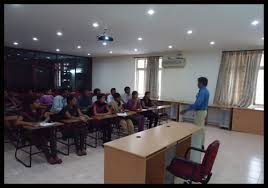## Alternative Models Of Computation

Published on Jan 03, 2023

### Abstract

The seminar aims at introducing various other forms of computation methods. Concepts of quantum computing ,DNA computing have been introduced and discussed . Particular algorithms (like the Shor's algorithm) have been discussed.

Solution of Traveling alesman problem using DNA computing has also been discussed . In ¯ne,the seminar aims opening windows to topics that may become tomorrow's mainstay in computer science.

Richard Feynman thought up the idea of a 'quantum computer', a computer that uses the e®ects of quantum mechanics to its advantage .Initially, the idea of a 'quantum computer' was primarily of theoretical interest only, but recent developments have bought the idea to foreground. To start with, was the invention of an algorithm to factor large numbers on a quantum computer, by Peter Shor ,from Bell labs . By using this algorithm, a quantum computer would be able to crack codes much more quickly than any ordinary (or classical) computer could.

In fact a quantum computer capable of performing Shor's algorithm would be able to break current cryptography techniques(like the RSA) in a matter of seconds. With the motivation provided by this algorithm, the quantum computing has gathered momentum and is a hot topic for research around the globe. Leonard M. Adleman solved an unremarkable computational problem with an exceptional technique.

He had used 'mapping' to solve TSP. It was a problem that an average desktop machine could solve in fraction of a second. Adleman, however took , seven days to find a solution. Even then his work was exceptional, because he solved the problem with DNA. It was a breakthrough and a landmark demonstration of computing on the molecular level.

In case of quantum computing and DNA computing ,both have two aspects.Firstly building a computer and secondly deploying the computer for solving problems that are tough to solve in the present domain of Von Neumann architecture. In the seminar we would consider the later.

### Shor's Algorithm

Shor's algorithm is based on a result from number theory. Which states : The function

f(a) = x pow a mod n

is a periodic function, where x and n are coprime . In the context of Shor's algorithm n is the number we wish to factor.

By saying we mean that their greatest common divisor is one.

If implemented, it will have a profound e®ect on cryptography, as it would compromise the security provided by public key encryption (such as RSA).We all know that the security lies in the 'hard' factoring problem. Shor's algorithm makes it simple using quantum computing techniques.

 Are you interested in this topic.Then mail to us immediately to get the full report. email :- contactv2@gmail.com